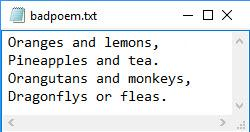# ftell

Current position

## Syntax

``position = ftell(fileID)``

## Description

example

````position = ftell(fileID)` returns the current location of the position pointer in the specified file. If the query is successful, then `position` is a zero-based integer that indicates the number of bytes from the beginning of the file.If the query is unsuccessful, then `position` is `-1`. ```

## Examples

collapse all

When you open a file, MATLAB® creates a pointer to indicate the current position within the file. Open the following `badpoem.txt` file and perform a read operation (which advances the position pointer). Then, query the final position in the file after the read operation.Use `fopen` to open the file. Then, use `ftell` to query the current position.

```fid = fopen('badpoem.txt'); ftell(fid)```
```ans = 0 ```

Using `fgetl`, read the first line and examine the current position after the read operation.

`tline1 = fgetl(fid) % read the first line `
```tline1 = 'Oranges and lemons,' ```
`ftell(fid)`
```ans = 20 ```

Read the second line and examine the current position.

`tline2 = fgetl(fid) % read the second line `
```tline2 = 'Pineapples and tea.' ```
`ftell(fid)`
```ans = 40 ```

Close the file.

`fclose(fid);`

## Input Arguments

collapse all

File identifier of an open file, specified as an integer. To open a file and obtain its valid identifier use the `fopen` function.

Data Types: `double`

## Version History

Introduced before R2006a

expand all